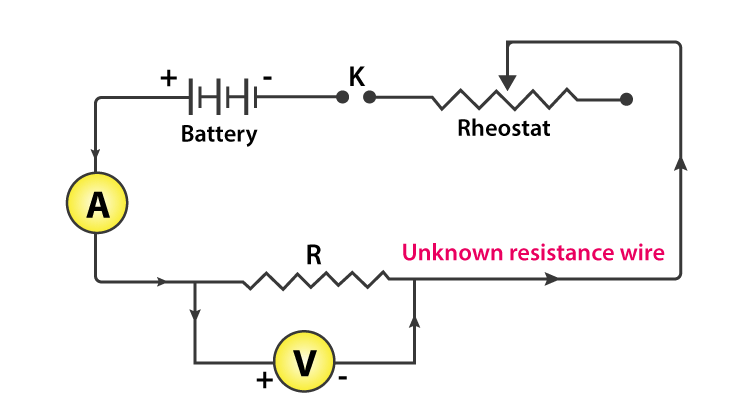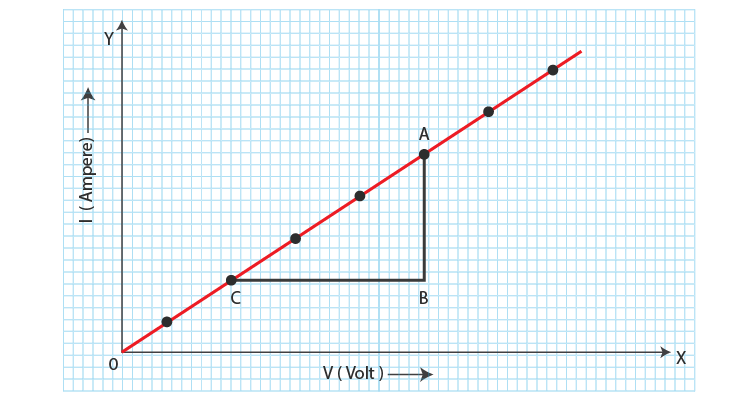Checkout JEE MAINS 2022 Question Paper Analysis : Checkout JEE MAINS 2022 Question Paper Analysis :

# To Determine Resistance Per Cm Of A Given Wire By Plotting A Graph For Potential Difference Versus Current

We know that resistance is a measure of the opposition to the flow of electricity in a circuit. The potential difference helps to understand the amount of energy transferred between two points in a circuit. In this session, let us learn to determine the resistance per cm of a given wire by plotting a graph for potential difference versus current.

## Aim

To determine the resistance per cm of a given wire by plotting a graph for potential difference versus current.

## Apparatus/Material Required

• A wire of unknown resistance
• Battery
• Voltmeter
• Milliammeter
• Rheostat
• Plug key
• Connecting wires
• Piece of sandpaper

## Circuit Diagram## Theory

According to Ohm’s law, the electric current flowing through a conductor is directly proportional to the potential difference across its ends, provided the physical state (pressure, temperature, and dimensions) of the conductor remains unchanged.

If I is the current flowing through the conductor and V is the potential difference across its end, then

$$\begin{array}{l}V\propto I\end{array}$$

and hence

V = RI

Where R is the constant of proportionality and is termed as the electrical resistance of the conductor. Resistance R depends on the dimensions and material of the conductor. The relationship between the resistance of a material and its length and area of the cross-section is given by the formula

$$\begin{array}{l}R=\rho \frac{l}{A}\end{array}$$

Where

$$\begin{array}{l}\rho\end{array}$$
is the specific resistance or resistivity and is a characteristic of the material of the wire.

## Procedure

1. Clean the ends of the connecting wire with the help of sandpaper to remove any insulating coating on them.
2. Connect the resistance, rheostat, battery, key, voltmeter, and ammeter as shown in the figure.
3. Make sure that the pointers in the voltmeter and milliammeter coincide with the zero mark on the measuring scale. If not, adjust the pointer to coincide with the zero mark by adjusting the screw provided at the base using a screwdriver.
4. Note the range and the least count of the given voltmeter and milliammeter.
5. Insert the key K and slide the rheostat to the end where the current flow is minimum.
6. Note the voltmeter and the milliammeter reading.
7. Remove the key K and allow the wire to cool. Again insert the key and slightly increase the voltage by moving the rheostat. Note down the milliammeter and voltmeter reading.
8. Repeat step 7 for four different adjustments of the rheostat. Document the readings in a tabular column.

## Observations

Range of ammeters = _____ mA to _____ mA

The Least count of ammeter = _____ mA

Range of voltmeter = _____ V to ____ V

The Least count of voltmeter = _____ V

The Least count of meter-scale = _____ m

Length of the given wire, l = _____ m

 S. No The applied potential difference (voltmeter reading V) Current flowing through the wire (Milliammeter Reading A)

## Calculations

1. Plot a graph between the potential difference across the wire V and the current I flowing through the wire as shown below.2. Determine the slope of the graph. The resistance of the given wire is then equal to the

Reciprocal of the slope.

From the graph, R = BC/AB = _____ Ω

3. Resistance per unit length of the wire = R/t = _____ Ωm–1

Error

$$\begin{array}{l}\frac{\Delta R}{R}=\frac{\Delta V}{V}+\frac{\Delta I}{I}=….\end{array}$$

Here, R is the resistance per unit length and ΔR is the estimated error. ΔV

and ΔI are the least count of voltmeter and ammeter respectively.

## Result

The potential difference across the wire varies linearly with the current.

The resistance per unit length of the wire is ( R ± ΔR) = _____ ± _____ Ωm–1).

## Viva Voice

1. State Ohm’s Law.

Ohm’s law states that the potential difference across an ideal conductor is proportional to the current through it. The constant of proportional is known as the resistance R. Ohm’s law is given by V = IR.

2. Which are the factors on which the resistance of a conductor depends on?

The resistance of a conductor depends on the following factors:

• Resistivity
• Length
• Temperature
• Cross-sectional area

3. What is a rheostat?

A rheostat is a variable resistance that is used to control the current.

4. What is the shape of a V vs I graph for a linear resistor?